Wednesday 21st October 2020CBSE Guess > Papers > Important Questions > Class XII > 2009 > Maths > Mathematics By Anil Kumar Tondak

CBSE CLASS XII

Relations & Functions

Q. 1. Show that the relation R in the set A =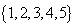given by R=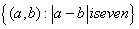,
is an equivalence relation.

Q. 2. Show that the relation R in R defined by R=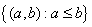, is reflexive and transitive
but not symmetric.

Q. 3. Let A= R ─{3} and B = R─{1} , Consider the function ƒ: A ® B defined by ƒ (x) =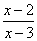.
Show that ƒ is one–one and onto
Q. 4. If ƒ: R ® R be given by ƒ (x) = (3-x3)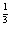, find the value of ƒoƒ (x)

Q. 5. Consider  ƒ : R ® R given by f(x) = 4x+3, show that ƒ is invertible . Find the inverse of ƒ.

Q. 6. Show that binary operation a * b =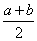" a,b ò N is commutative but not associative.
Q. 7. Show that  ƒ: N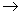N defined by  ƒ (x) =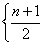, if n is odd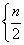, if n is even is many-one onto function.

Q. 8. Show that  ƒ : R –{0}R–{0} given by  ƒ (x) = 3/x is invertible and it is inverse of itself.

Q. 9. On the set M = A (x) =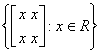of 2 ´ 2 matrices, find the identity element for the multiplication of matrices as a binary operation. Also, find the inverse of an element of M.

Q. 10. Let  ƒ (x) = [x] and g(x) = êx ê find  goƒ (-5/3) –  ƒog (-5/3)

Q. 10. Show that the function ƒ: RR defined by  ƒ (x) = 3x3 + 5 for x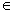R is a bijection.

Q. 12. Show that the relation R on the set R of all real numbers, defined as R = { (a, b): a £ b2 } is neither reflexive nor symmetric nor transitive.

Q. 13. Show that the function ƒ:N ® Ngiven by ƒ (1) = ƒ (2) =1 and ƒ (x) = x-1,for every x > 2 is  onto but not one-one.

Prepared By Anil Kumar Tondak
M.Sc.(Maths), M.Ed., M.A.(Eco),M.B.A.(Mkt)
Mob No. 9811363962

 Maths